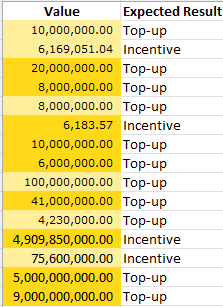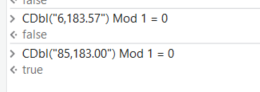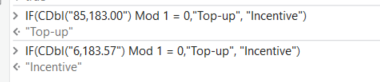# How to identify and classify the round value and not round value in Excel or DataTable

Hi all,

I have a case to identify and classify the value in DataTable or Excel between round value and non-round value.
This is the screenshot with the expected result from my case.
Hope you can help me to solve it, thank you very much@Gece_De
Welcome to the forum

One of more options is to use the Modulo function for a check:Along with the IF Function we can calculate the label:Hi,

Another approach:

``````v = Decimal.Parse(CurrentRow("Value").ToString,System.Globalization.NumberStyles.Any)
``````

Then

``````v-Math.Round(v,MidpointROunding.AwayFromZero)=0
``````

Sample20230313-9L.zip (3.2 KB)

Regards,